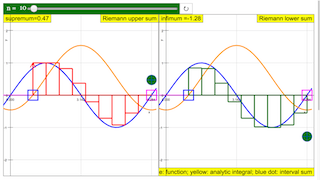Description

Riemann integral

In two dimensions the Rieman integral determines the area between the x axis and the function y = f(x) in an interval x1 < x < x2 by nesting it between two approximative sums. Both are constructed by a series of rectangles with intervals along the x axis. For the upper sum the approximative value of y in each interval is equal to the largest value in the interval (its supremum);  for the lower sum it is equal to the smalles value (infimum). The Riemann integral exists, when both sums converge with decreasing interval width, and when they converge to the same limit.

The definition is not identical to the classical rectangle algorithm, where the value of the function is equal to the value at the beginning of the interval (more generally: always at the same point in the interval). For "well behaved" functions there will be no difference in results, yet the Riemann definition is more generally applicable.

The approximative calculation of the Riemann integral is shown for the example of a sine function (blue). Its definite integral is to be calculated in the range x2 - x1. The initial value x1 is defined by a slider, the end point x2 by drawing the red point with the mouse. The yellow curve is the analytic solution cos x - cos x1.

The number of intervals (n - 1) is defined by slider n. Reset defines 1 < x < 4 and n = 10 (9 intervals) .

The left window shows in red the approximation by the supremum series, with the blue point as the sum; the function always lies below the rectangle. The right window shows the approximation by the infimum series; the function always lies above the rectangle.

Correspondingly the upper sum of the rectangles is always higher than the analytic integral, while the lower sum is always lower - for finite interval widths. With decreasing interval widths both converge to the same value, the Riemann integral.

Experiments

E1: Start with the default setting: x1 = 1; x2 = 4;  n = 10.

Verify that both graphs really limit the intervals in the y direction by the highest, respectively the lowest value in the interval (observe the sign of the function itself!). Consider the systematic deviation of the sum from the analytic solution.

E2: Compare the construction with the classical rectangle (step) algorithm.

E4: Increase n and observe the process of convergence to the limit value as the interval width decreases.

E4: Draw the end point at a large number for n and observe how the intervals limit lines "paint" the area under the curve. You will not visibly recognize a difference between sum and integral.

E5: Change the initial point with the slider. Draw the end point beyond the initial point and control if the result is still correct.

Translations

Code Language Translator RunSoftware Requirements

SoftwareRequirements

 Android iOS Windows MacOS with best with Chrome Chrome Chrome Chrome support full-screen? Yes. Chrome/Opera No. Firefox/ Samsung Internet Not yet Yes Yes cannot work on some mobile browser that don't understand JavaScript such as..... cannot work on Internet Explorer 9 and below

CreditsDieter Roess - WEH-Foundation; Fremont Teng; Loo Kang Wee

[text]

Riemann Integral JavaScript Simulation Applet HTML5

Instructions

N Slider

Toggling the slider will set the number of rectangles drawn.

Draggable Box

Drag the blue/magenta boxes to set the two coordinates respectively.

Note that moving a pair on the graph will directly affect the other graph as well.

Toggling Full Screen

Double clicking anywhere in the panel will toggle full screen.

Reset Button

Resets the simulation.

Research

[text]

[text]

[text]

Testimonials (0)

There are no testimonials available for viewing. Login to deploy the article and be the first to submit your review!